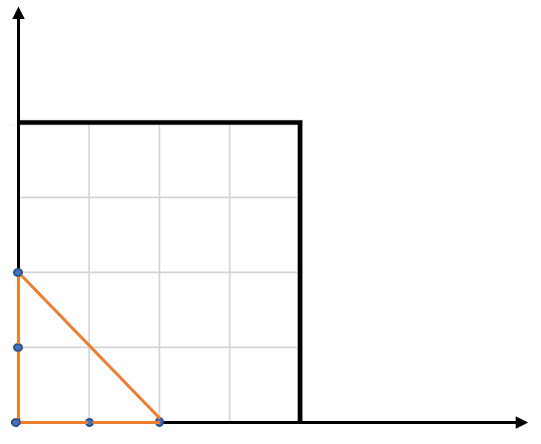##### Welcome to Subscribe On Youtube

Formatted question description: https://leetcode.ca/all/812.html

# 812. Largest Triangle Area (Easy)

You have a list of points in the plane. Return the area of the largest triangle that can be formed by any 3 of the points.

Example:
Input: points = [[0,0],[0,1],[1,0],[0,2],[2,0]]
Output: 2
Explanation:
The five points are show in the figure below. The red triangle is the largest.Notes:

• 3 <= points.length <= 50.
• No points will be duplicated.
•  -50 <= points[i][j] <= 50.
• Answers within 10^-6 of the true value will be accepted as correct.

Companies:

Related Topics:
Math

## Solution 1.

• class Solution {
public double largestTriangleArea(int[][] points) {
double largestArea = 0;
int length = points.length;
for (int i = 0; i < length; i++) {
int[] point1 = points[i];
for (int j = i + 1; j < length; j++) {
int[] point2 = points[j];
for (int k = j + 1; k < length; k++) {
int[] point3 = points[k];
if (sameLine(point1, point2, point3))
continue;
double area = getArea(point1, point2, point3);
largestArea = Math.max(largestArea, area);
}
}
}
return largestArea;
}

public boolean sameLine(int[] point1, int[] point2, int[] point3) {
int delta1X = point2 - point1, delta1Y = point2 - point1;
int delta2X = point3 - point2, delta2Y = point3 - point2;
return delta1X * delta2Y == delta2X * delta1Y;
}

public double getArea(int[] point1, int[] point2, int[] point3) {
double side1 = getDistance(point1, point2);
double side2 = getDistance(point2, point3);
double side3 = getDistance(point3, point1);
double halfPerimeter = (side1 + side2 + side3) / 2;
double area = Math.sqrt(halfPerimeter * (halfPerimeter - side1) * (halfPerimeter - side2) * (halfPerimeter - side3));
return area;
}

public double getDistance(int[] point1, int[] point2) {
return Math.sqrt(((double) point2 - (double) point1) * ((double) point2 - (double) point1) + ((double) point2 - (double) point1) * ((double) point2 - (double) point1));
}
}

############

class Solution {
public double largestTriangleArea(int[][] points) {
double ans = 0;
for (int[] p1 : points) {
int x1 = p1, y1 = p1;
for (int[] p2 : points) {
int x2 = p2, y2 = p2;
for (int[] p3 : points) {
int x3 = p3, y3 = p3;
int u1 = x2 - x1, v1 = y2 - y1;
int u2 = x3 - x1, v2 = y3 - y1;
double t = Math.abs(u1 * v2 - u2 * v1) / 2.0;
ans = Math.max(ans, t);
}
}
}
return ans;
}
}

• // OJ: https://leetcode.com/problems/largest-triangle-area/
// Time: O(N^3)
// Space: O(1)
// Ref: https://leetcode.com/problems/largest-triangle-area/solution/
class Solution {
private:
double area (vector<int> &a, vector<int> &b, vector<int> &c) {
return .5 * abs(a*b + b*c + c*a
-a*b - b*c - c*a);
}
public:
double largestTriangleArea(vector<vector<int>>& points) {
double ans = 0;
for (int i = 0, N = points.size(); i < N; ++i) {
for (int j = i + 1; j < N; ++j) {
for (int k = j + 1; k < N; ++k) {
ans = max(ans, area(points[i], points[j], points[k]));
}
}
}
return ans;
}
};

• class Solution:
def largestTriangleArea(self, points: List[List[int]]) -> float:
ans = 0
for x1, y1 in points:
for x2, y2 in points:
for x3, y3 in points:
u1, v1 = x2 - x1, y2 - y1
u2, v2 = x3 - x1, y3 - y1
t = abs(u1 * v2 - u2 * v1) / 2
ans = max(ans, t)
return ans

############

class Solution:
def largestTriangleArea(self, points):
"""
:type points: List[List[int]]
:rtype: float
"""
res = 0
N = len(points)
for i in range(N - 2):
for j in range(i + 1, N - 1):
for k in range(i + 2, N):
(x1, y1), (x2, y2), (x3, y3) = points[i], points[j], points[k]
res = max(res, 0.5 * abs(x1 * (y2 - y3) + x2 * (y3 - y1) + x3 * (y1 - y2)))
return res

• func largestTriangleArea(points [][]int) float64 {
ans := 0.0
for _, p1 := range points {
x1, y1 := p1, p1
for _, p2 := range points {
x2, y2 := p2, p2
for _, p3 := range points {
x3, y3 := p3, p3
u1, v1 := x2-x1, y2-y1
u2, v2 := x3-x1, y3-y1
t := float64(abs(u1*v2-u2*v1)) / 2.0
ans = math.Max(ans, t)
}
}
}
return ans
}

func abs(x int) int {
if x < 0 {
return -x
}
return x
}